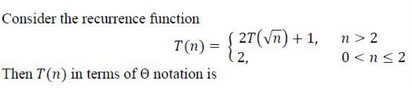Skip to content
Related Articles
GATE | GATE-CS-2017 (Set 2) | Question 56
• Last Updated : 16 Feb, 2017(A) θ(log log n)
(B) θ(log n)
(C) θ(sqrt(n))
(D) θ(n)

Answer: (B)

Explanation:

```
T(n) = 2T(√n) + 1

Let n = 2m

==> T(2m) = 2T(2m/2) + 1

Let S(m) = T(2m)
==> S(m/2) = T(2m/2)

Thus above equation will be : S(m) = 2S(m/2) + 1
Applying master’s theorem S(m) = m
Thus : T(n) = Θlog(n) (since n = 2m)
```

This question is duplicate of http://quiz.geeksforgeeks.org/algorithms-analysis-of-algorithms-question-17-2/

Quiz of this Question

Attention reader! Don’t stop learning now. Learn all GATE CS concepts with Free Live Classes on our youtube channel.

My Personal Notes arrow_drop_up